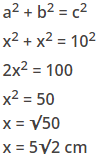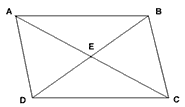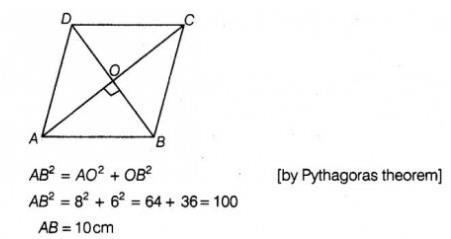# Test: Parallelograms Diagonal Properties

## 5 Questions MCQ Test Mathematics (Maths) Class 9 | Test: Parallelograms Diagonal Properties

Description
This mock test of Test: Parallelograms Diagonal Properties for Class 9 helps you for every Class 9 entrance exam. This contains 5 Multiple Choice Questions for Class 9 Test: Parallelograms Diagonal Properties (mcq) to study with solutions a complete question bank. The solved questions answers in this Test: Parallelograms Diagonal Properties quiz give you a good mix of easy questions and tough questions. Class 9 students definitely take this Test: Parallelograms Diagonal Properties exercise for a better result in the exam. You can find other Test: Parallelograms Diagonal Properties extra questions, long questions & short questions for Class 9 on EduRev as well by searching above.
QUESTION: 1

Solution:
QUESTION: 2

### The length of the diagonal of the square is 10 cm. The area of the square is:​

Solution:

If side of square is 'a', then √2a = 10 Squaring both sides, 2a=100 a= 50 Area is 50 sqcm.

QUESTION: 3

### The diagonal of a square is 10 cm. Its area is:​

Solution:

Let x = the length of a side of the squareSo area of square = x2 = 50cm2

QUESTION: 4

ABCD is a parallelogram E is the midpoint of _______.Solution:
QUESTION: 5

The diagonals of rhombus are 12 cm and 16 cm. The length of the side of rhombus is:

Solution:

We know that, the diagonals of a rhombus are perpendicular bisector of each other.
Given,  AC = 16 cm and BD = 12 cm        [let]
∴ AO = 8cm, SO = 6cm
and  ∠AOB = 90°
In right angled ∠AOB,